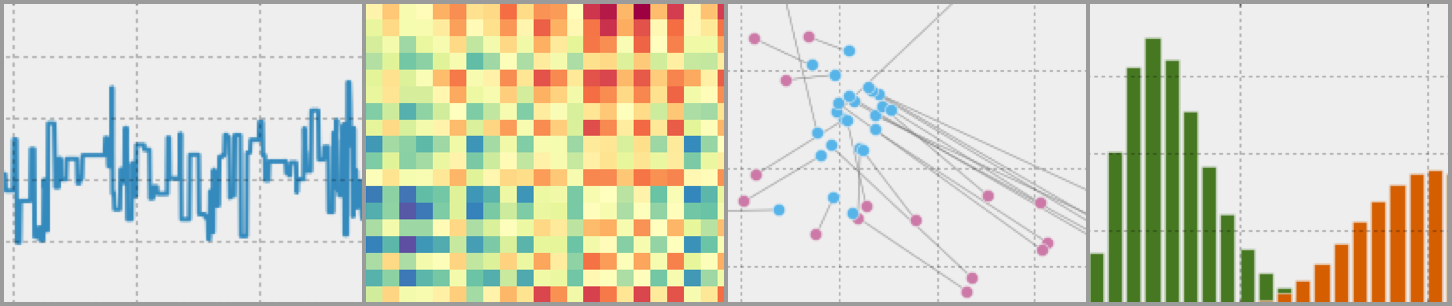# Bayesian Modelling In Python

A python tutorial on bayesian modeling techniques (PyMC3)
Alternatives To Bayesian Modelling In Python
Bayesian Modelling In Python2,202
6 years agoJupyter Notebook
A python tutorial on bayesian modeling techniques (PyMC3)
Bayesian Stats Modelling Tutorial601
a year ago14mitJupyter Notebook
How to do Bayesian statistical modelling using numpy and PyMC3
Sklearn Bayes439
2 years ago20mitJupyter Notebook
Python package for Bayesian Machine Learning with scikit-learn API
3 years ago3Jupyter Notebook
Code for a tutorial on Bayesian Statistics by Allen Downey.
Scipy2014_tutorial281
4 years ago1Jupyter Notebook
Tutorial: Bayesian Statistical Analysis in Python
Bayes_computing_course208
2 years ago1mitJupyter Notebook
Trieste170
5 days ago25January 31, 202393apache-2.0Python
A Bayesian optimization toolbox built on TensorFlow
Tutorials168
2 years agoHTML
Tutorials on phylogenetic and phylogenomic inference
Mcmc_pydata_london_201975
3 years ago2mitJupyter Notebook
PyData London 2019 Tutorial on Markov chain Monte Carlo with PyMC3
Tutorial63
2 years ago3gpl-3.0Jupyter Notebook
Tutorial on Bayesian tests for Machine Learning
Alternatives To Bayesian Modelling In Python
Select To Compare

Alternative Project Comparisons

# Bayesian Modelling in PythonWelcome to "Bayesian Modelling in Python" - a tutorial for those interested in learning how to apply bayesian modelling techniques in python (PYMC3). This tutorial doesn't aim to be a bayesian statistics tutorial - but rather a programming cookbook for those who understand the fundamental of bayesian statistics and want to learn how to build bayesian models using python. The tutorial sections and topics can be seen below.

### Contents

• Introduction

• Motivation for learning bayesian statistics
• Section 1: Estimating model parameters

• Frequentist technique for estimating parameters of a poisson model (Optimization routine)
• Bayesian technique for estimating parameters of a poisson model (MCMC)
• Section 2: Model checking & comparison

• Posterior predictive check
• Bayes factor
• Section 3: Hierarchal modeling

• Model pooling (separate models)
• Partial pooling (hierarchal models)
• Shrinkage effect of partial pooling
• Section 4: Bayesian regression

• Bayesian fixed effects poisson regression
• Bayesian mixed effects poisson regression
• Section 5: Bayesian survival analysis

• Survival model theory
• Cox proportional hazard model
• Section 6: Bayesian A/B tests

• Bayesian test of proportions
• Bayesian t-test (BEST)

### Contributions

• All contributions are more than welcome. They can be minor (spelling, better explanations, improved code/charts) or major (contribute a full section).
• If you would like to contribute, please create a pull request in GitHub. Happy to discuss ideas before you begin working on the addition.
• I would especially welcome any contributions that address: survival analysis, mixture models, time series models or A/B experiments.
• If you're not familiar with GitHub - please email me at [email protected].

### Motivation for learning bayesian statistics

Statistics is a topic that never resonated with me throughout university. The frequentist techniques that we were taught (p-values etc) felt contrived and ultimately I turned my back on statistics as a topic that I wasn't interested in.

That was until I stumbled upon Bayesian statistics - a branch to statistics quite different from the traditional frequentist statistics that most universities teach. I was inspired by a number of different publications, blogs & videos that I would highly recommend any newbies to bayesian stats to begin with. They include:

I created this tutorial in the hope that others find it useful and it helps them learn Bayesian techniques just like the above resources helped me. I hope you find it useful and I'd welcome any corrections/comments/contributions from the community.

### Note

This tutorial is actively being worked on. I'm keen to get feedback and welcome ideas/contributions.

Popular Bayesian Projects
Popular Tutorials Projects
Popular Machine Learning Categories
Related Searches

Get A Weekly Email With Trending Projects For These Categories
No Spam. Unsubscribe easily at any time.
Python
Jupyter Notebook
Tutorials
Statistics
Bayesian
Bayesian Statistics